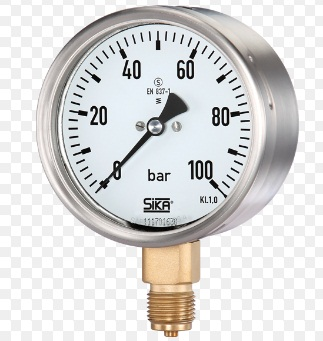# Basics of Pressure Scales### Introduction

There are three pressure scales used for measuring pressure.

• The Absolute pressure scale
• The Gauge pressure scale
• The Vacuum pressure scale

In the pressure gauges shown in Figure all pressure gauges show a reading of 20, but their values of pressure are all different.

Gauge A               Shows a pressure of 20 pounds per square inch absolute

Gauge B               Shows a pressure of 20 pounds per square inch gauge

Gauge C               Shows a pressure of 20 inches Mercury Vacuum

In order to understand why these pressure gauges  represent different values of  pressure,  you have to understand the concept of Absolute pressure,  Gauge pressure and Vacuum pressure.Pressure gauges with different pressure scales

### Absolute Pressure scale

Absolute pressure is when the pressure is measured using absolutely no pressure as a starting point.

Air, which is a mixture of gases, has weight.  The force of gravity attracts the air.  At sea-level the ‘standard’ weight of the earth’s atmosphere exerts a pressure of 14.7 PSI  Atmospheric pressure.

Since we all are subjected to the pressure of the earth’s atmosphere all the time, we may think of it as no pressure at all and consider the pressure of the earth’s atmosphere to be zero.  Yet it is a very real pressure.  If we move all the air out of a closed chamber, there would be no pressure within the chamber, so the pressure would be absolutely zero.

The absolute pressure scale uses absolute zero pressure as its starting point.  Absolute pressure is the complete absence of pressure, even Atmospheric pressure.

Pressure readings taken from an absolute pressure scale are expressed as pounds per square inch absolute (PSIA).

An unconnected pressure gauge that uses absolute pressure scale will read 14.7 PSIA if it is accurate.

Absolute pressure = Gauge pressure  +  Atmospheric pressure.An unconnected pressure gauge with absolute pressure scale

### Gauge Pressure scale

Gauge pressure is pressure measured using atmospheric pressure as a starting point and calling this pressure ZERO.

Most pressures measured in Gas/ Oil processing are  gauge pressures.  The gauge pressure uses atmospheric pressure as the starting point for all measurements.  This means that the gauge scale starts at zero even though there is still atmospheric pressure on it.

An unconnected pressure gauge using gauge pressure scales will read zero if it is accurate.

Gauge pressure = Absolute pressure – Atmospheric pressureAn unconnected pressure gauge using gauge pressure

### Vacuum Pressure scale

A vacuum is a space that contains no air.  In hydrocarbon processing plants, vacuum means pressure lower than atmospheric pressure.  Vacuum pressure is commonly measured in inches of mercury.  A reading of zero on the mercury vacuum gauge is equal to atmospheric pressure.  The vacuum scale is used to measure pressures in pipes or vessels which are below atmospheric pressures.

An unconnected pressure gauge with a vacuum scale will read zero.An unconnected pressure gauge with a vacuum pressure scale

Scroll to Top